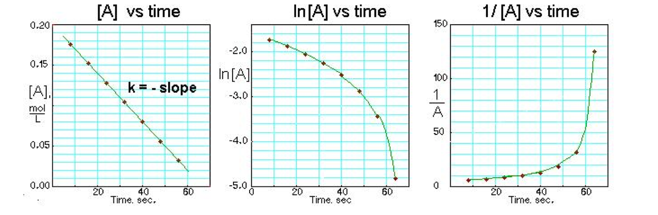# Solution: The three plots were done based on a chemical reaction. Based on their sketches answer each of the following questions.  What is the order of the reaction?

###### Problem

The three plots were done based on a chemical reaction. Based on their sketches answer each of the following questions.  What is the order of the reaction?